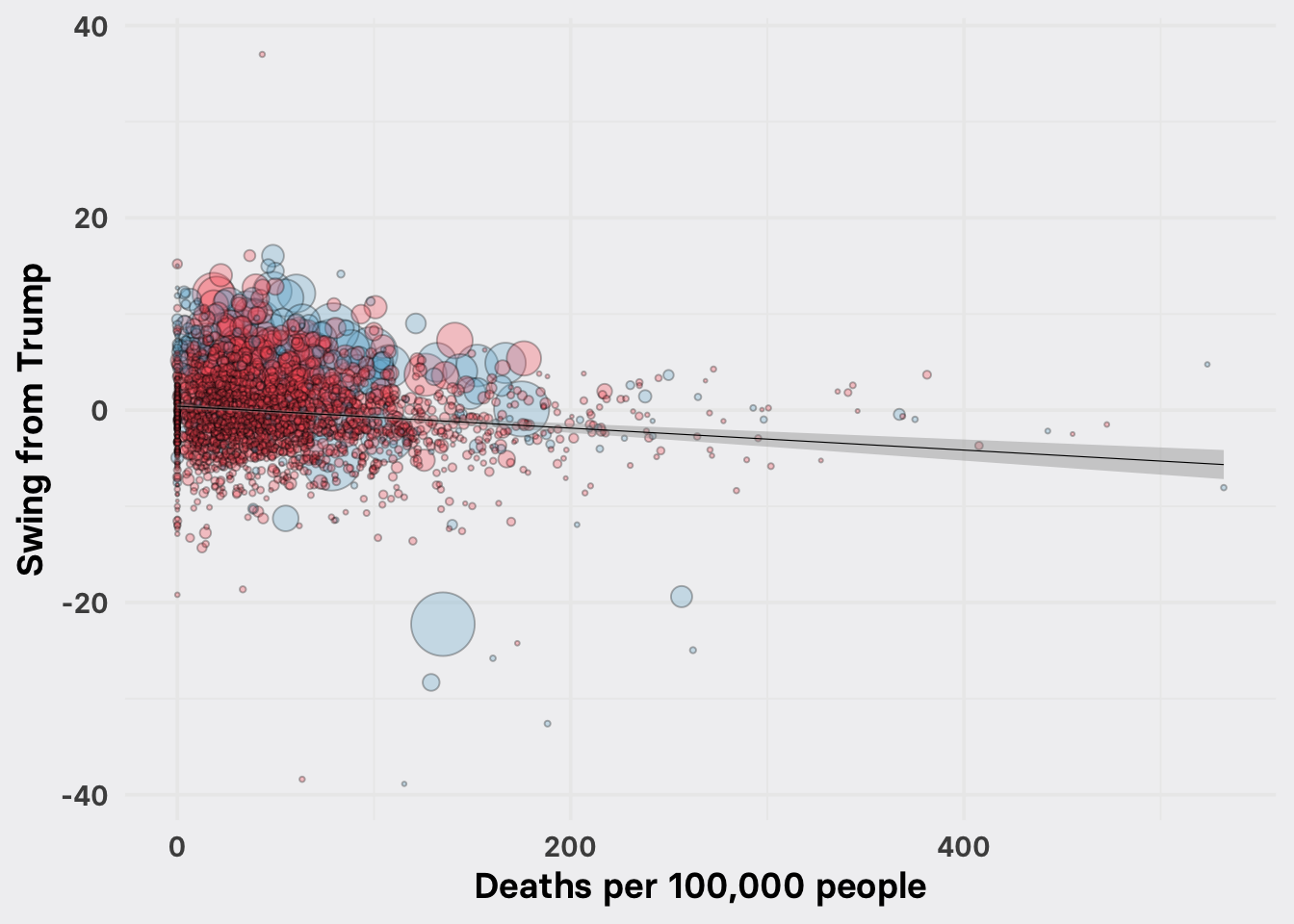Data and R code behind the analysis referenced in this Nov. 11, 2020 BuzzFeed News post discussing the extent to which death rates from COVID-19 and surges in unemployment related to pandemic lockdowns could explain swings in voter preference at the county level between the 2016 and 2020 presidential elections.

County-level election returns data for 2020 is from Decision Desk HQ, complete to 10 am Eastern, Nov. 10; data for the 2016 election is from the MIT Election Data + Science Lab. Data on cumulative COVID-19 death rates by county to Nov. 2 is from the New York Times (and USAFacts for counties within New York City and in the Kansas City area). County-level data on unemployment rates is from the Bureau of Labor Statistics; because this data is not seasonally corrected, we calculated the annual change in unemployment rate over the 12 months to the most recent month available, September 2020. Counts in many counties for the 2020 election have not yet finished. To minimize any biases caused by counties with large numbers of uncounted votes, the analysis includes only counties that where the vote count exceeded 95% of the turnout predicted by Decision Desk HQ before the election.

``````# required packages
library(tidyverse)
library(tidymodels)

# load and process election results data
mutate(swing = dem_vs_prev * 100,
lead = case_when(dem_margin < 0 ~ "Trump",
dem_margin > 0 ~ "Biden")) %>%
filter(!is.na(swing) & vote_prop_est > 0.95) %>%

# load county level unemployment data

# joins
covid_results_unem <- inner_join(covid,results, by = c("fips_county" = "geoid")) %>%
select(-state.x,-county_name) %>%
rename(state = state.y) %>%
inner_join(unem) %>%

### Scatter plot of COVID-19 death rate vs. swing

``````ggplot(covid_results_unem, aes(x = deaths_per_100k, y = swing, size = total_votes_2020)) +
geom_point(shape = 21, stroke = 0.5, color = "black", alpha = 0.3, aes(fill = lead)) +
geom_smooth(size = 0.2, method = "lm", se = TRUE, color = "black") +
scale_size_area(max_size = 15) +
scale_fill_manual(values = c("#74b3d3","#fb6066"), name = "") +
xlab("Deaths per 100,000 people") +
ylab("Swing from Trump") +
theme_minimal(base_family = "Basier Square SemiBold", base_size = 14) +
theme(legend.position = "none",
panel.background = element_rect(fill = "#f1f1f2", size = 0),
plot.background = element_rect(fill = "#f1f1f2", size = 0))``````On this chart, each circle is a county, scaled by the number of votes counted in the 2020 election; counties are colored blue if Biden leads in the count, red if Trump leads.

The swing is the difference in the percentage point margin between Donald Trump and Hillary Clinton in 2016 and the percentage point margin between Donald Trump and Joe Biden in 2020. A positive swing means that voters shifted away from Trump in 2020; a negative swing means they shifted toward him in 2020.

The trend line shows that, overall, voters seemed to swing slightly to Trump in the counties with higher COVID-19 death rates. The shaded gray ribbon shows the standard error of the linear trend line.

### Scatter plot of change in the unemployment rate vs. swing

``````ggplot(covid_results_unem, aes(x = points_change_unem_rate, y = swing, size = total_votes_2020)) +
geom_point(shape = 21, stroke = 0.3, color = "black", alpha = 0.5, aes(fill = lead)) +
geom_smooth(size = 0.2, method = "lm", se = TRUE, color = "black") +
scale_size_area(max_size = 15) +
scale_fill_manual(values = c("#74b3d3","#fb6066"), name = "") +
xlab("Change in unemployment rate") +
ylab("Swing from Trump") +
theme_minimal(base_family = "Basier Square SemiBold", base_size = 14) +
theme(legend.position = "none",
panel.background = element_rect(fill = "#f1f1f2", size = 0),
plot.background = element_rect(fill = "#f1f1f2", size = 0))``````